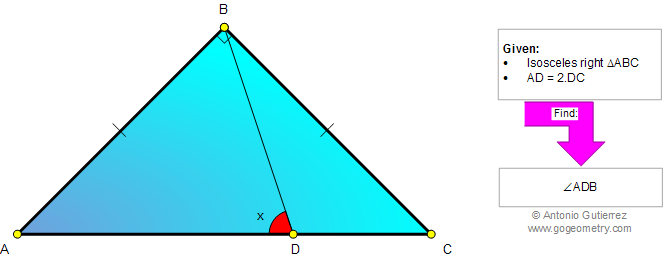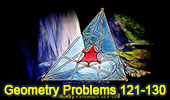# Problem 858: Isosceles Right Triangle, Cevian, Ratio 2:1, 45 Degrees, Congruence, Angles, Auxiliary Lines. Level: High School, SAT Prep, College geometry

 The figure shows an isosceles right triangle ABC with a point D on AC. If AD = 2 DC, find the measure of angle ADB. Try to use elementary geometry (no trigonometry).See More Geometry Problems:Geometry Problem 859 Isosceles Right Triangle, Angles,18, 45 Degrees, Congruence, Perpendicular bisector. Geometry Problem 857 Triangle, Median, Midpoint, Angles, 30, 45 Degrees, Congruence, Auxiliary Lines. Geometry Problem 856 Quadrilateral, Diagonals, Triangle, Angles, 30 degrees, Congruence, Auxiliary lines.
 Home | Search | Geometry | Problems | All Problems | Open Problems | Visual Index | 10 Problems | 851-860 | Triangles | Congruence | Angles | 45 degrees | Isosceles Triangle | Email | Solution / comment | By Antonio Gutierrez